# Class 9 RD Sharma Solutions – Chapter 13 Linear Equation in Two Variable- Exercise 13.3 | Set 1

• Last Updated : 30 Apr, 2021

### Question 1: Draw the graph of each of the following linear equations in two variables:

(i) x + y = 4

(ii) x – y = 2

(iii) -x + y = 6

(iv) y = 2x

(v) 3x + 5y = 15

(vi)(vii)(viii) 2y = -x +1

Solution:

(i) Given:

x + y = 4

or

y = 4 – x,

Now find values of x and y:

By putting x = 0 ⇒ y = 4

By putting x = 4 ⇒ y = 0

On the Graph: Mark points (0, 4) and (4, 0) on the graph and join them.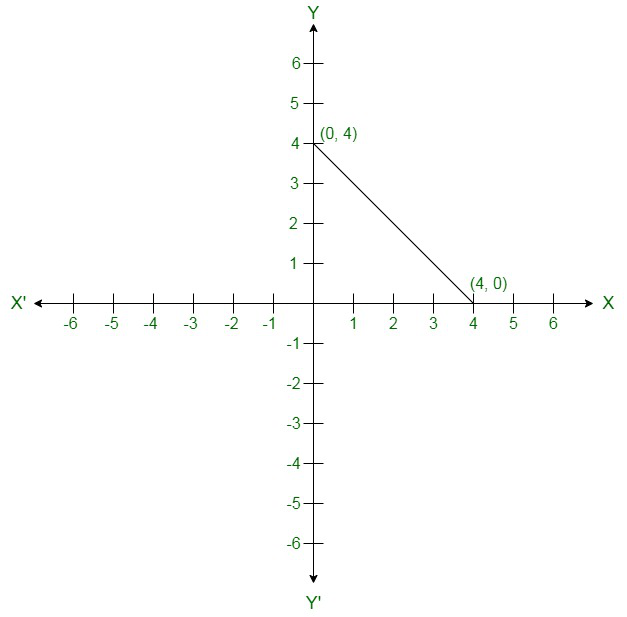(ii) Given:

x – y = 2

Thus,

y = x – 2

By putting x = 0 ⇒ y = – 2

By putting x = 2 ⇒ y = 0

On the graph: Mark points (0, -2) and (2, 0) on the graph and join them.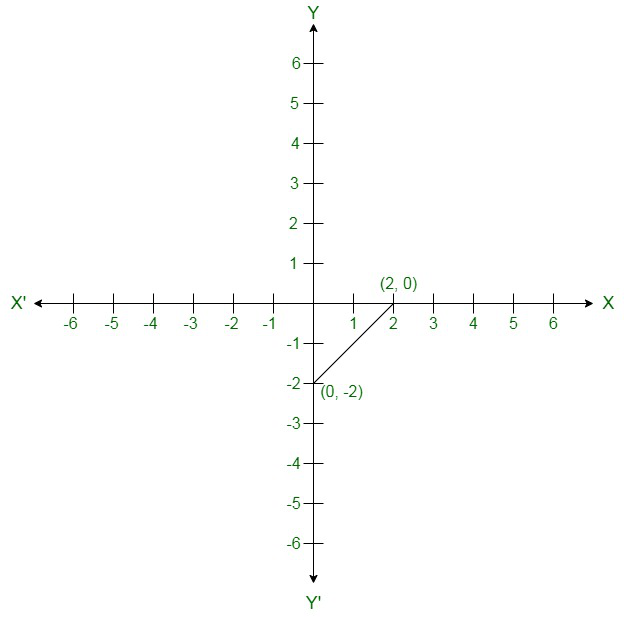(iii) Given:

– x + y = 6

Thus,

y = 6 + x

By putting x = 0 ⇒ y = 6

By putting x = -6 ⇒ y = 0

On the graph: Mark points (0, 6) and (-6, 0) on the graph and join them.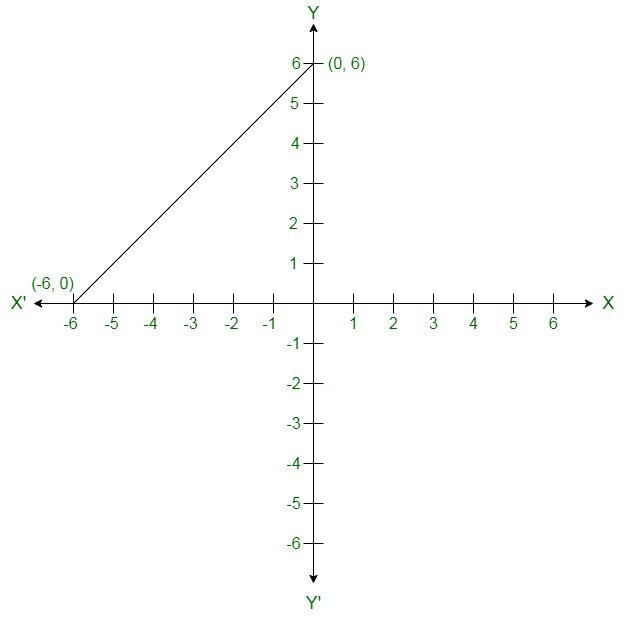(iv) Given:

y = 2x

After putting x = 1 ⇒ y = 2

Now put x = 3 ⇒ y = 6

On the graph: Mark points (1, 2) and (3, 6) on the graph and join them.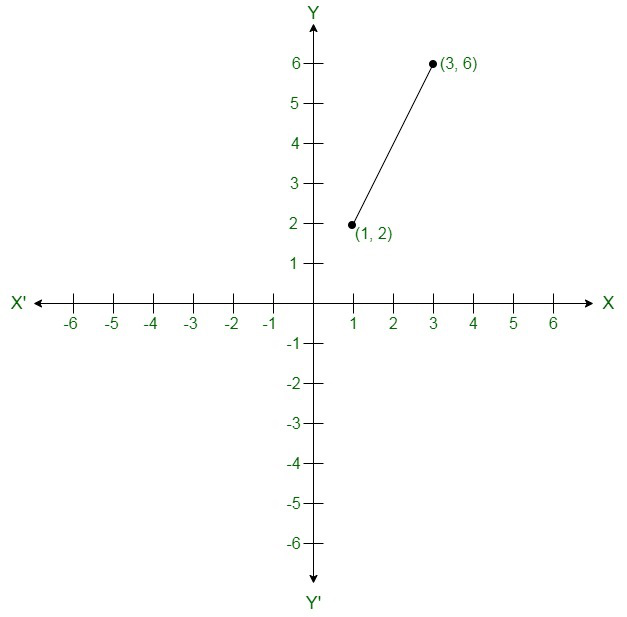(v) Given:

3x + 5y = 15

Or

5y = 15 – 3x

By putting x = 0 ⇒ 5y = 15 ⇒ y =3

By putting x = 5 ⇒ 5y = 0 ⇒ y = 0

On the graph: Mark points (0, 3) and (5, 0) on the graph and join them.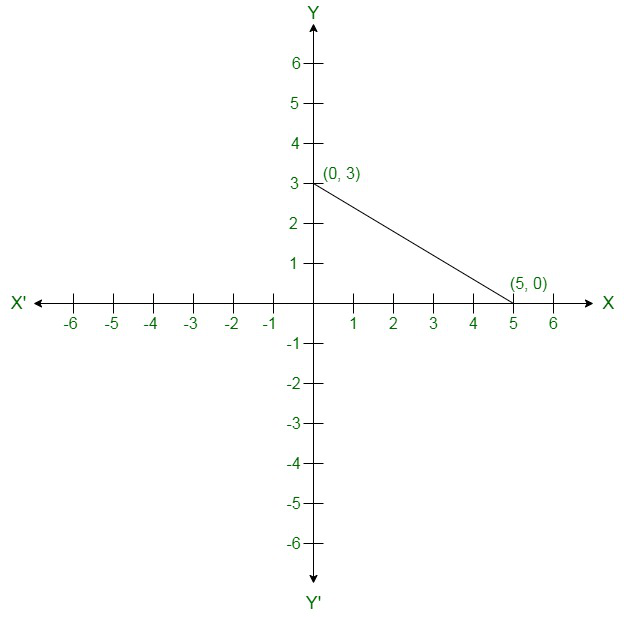(vi) Given:3x – 2y = 12By putting x = 0 ⇒ y = -6

By putting x = 4 ⇒ y = 0

On the graph: Mark points (0, -6) and (4, 0) on the graph and join them.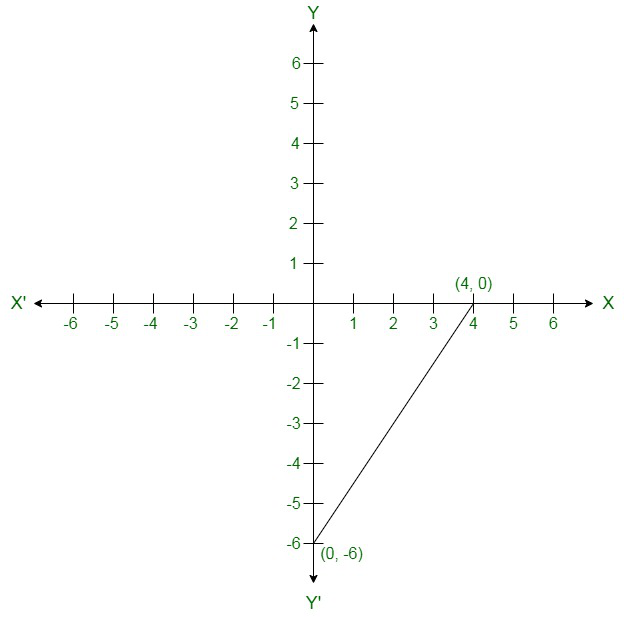(vii) Given:x – 2 = 3(y – 3)

x – 2 = 3y – 9

x = 3y – 7

Now,

By putting x = 5 in x = 3y – 7

y = 4

By putting x = 8 in x = 3y – 7,

y = 5

On the graph: Mark points (5, 4) and (8, 5) on the graph and join them.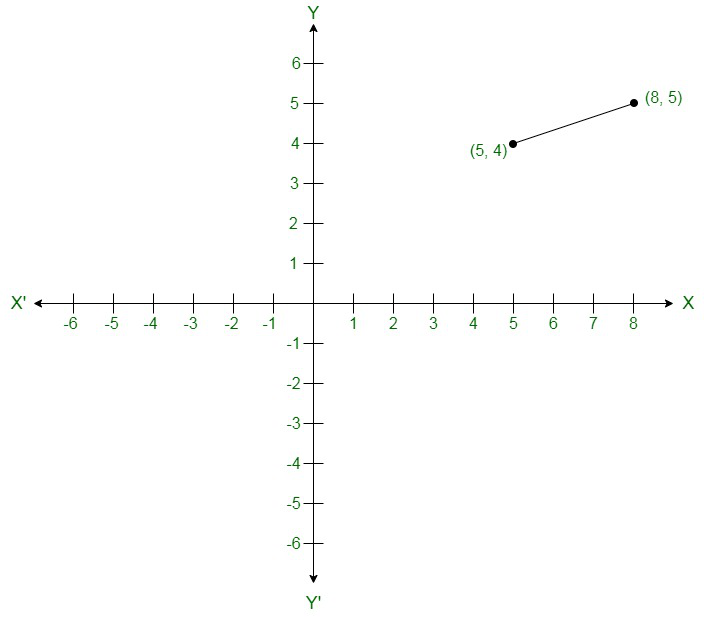(viii) Given:

2y = – x + 1

2y = 1 – x

Now,

By putting x = 1 in 2y = 1 – x,

we get;

y = 0

Again, By putting x = 5 in 2y = 1 – x,

we get;

y = -2

On the graph:

Mark points (1, 0) and (5, -2) on the graph and join them.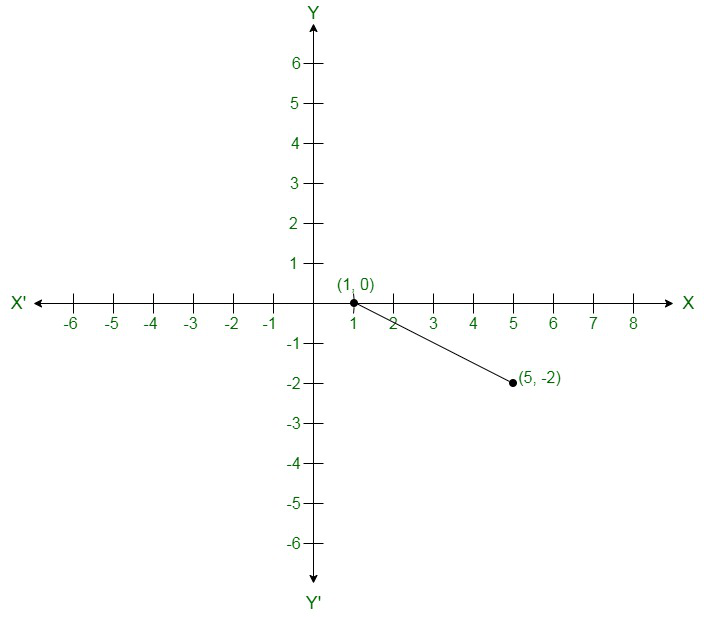### Question 2: Give the equations of two lines passing through (3, 12). How many more such lines are there, and why?

Solution:

Since here, a = 3 and b = 12 is the solution of the equations.

Thus, we have to find the set of any two equations which will satisfy this point.

Assume 4a – b = 0 and 3a – b + 3 = 0 be the set of lines which are passing through (3, 12).

As we know that – infinite lines can be pass through a point.

Hence, there are infinite lines passing through (3, 12).

### Question 3: A three-wheeler scooter charges Rs 15 for first kilometre and Rs 8 each for every subsequent kilometre. For a distance of x km, an amount of Rs y is paid. Write the linear equation representing the above information.

Solution:

Consider, the total fare for covering the distance of ‘x’ km is given by Rs y

According to the problem given:

y = 15 + 8(x – 1)

y = 15 + 8x – 8

y = 8x + 7

Thus, the above equation represents the linear equation for the given data.

### Question 4: A lending library has a fixed charge for the first three days and an additional charge for each day thereafter. Aarushi paid Rs 27 for a book kept for seven days. If fixed charges are Rs x and per day charges are Rs y. Write the linear equation representing the above information.

Solution:

As given: Aarushi paid Rs 27, of which Rs. x for the first three days and Rs. y per day for 4 more days is given by

x + (7 – 3) y = 27

x + 4y = 27

Thus, the above equation represents the linear equation for the given data.

### Question 5: A number is 27 more than the number obtained by reversing its digits. lf its unit’s and ten’s digit are x and y respectively, write the linear equation representing the statement.

Solution:

Given:

The original number is 27 more than the number obtained by reversing its digits

Thus the given number can be shown by  10y + x.

Number produced by reversing the digits of the number is 10x + y.

As per the problem:

10y + x = 10x + y + 27

10y – y + x – 10x = 27

9y – 9x = 27

9 (y – x) = 27

y – x = 3

x – y + 3 = 0

Thus, the above equation represents the linear equation for the given data.

### Question 6: The Sum of a two-digit number and the number obtained by reversing the order of its digits is 121. If units and ten’s digit of the number are x and y respectively, then write the linear equation representing the above statement.

Solution:

Given: The number is 10y + x.

After reversing the digits of the number,

We get,

10x + y

Sum of the two numbers is 121. (As given in the problem)

10y + x + 10x + y = 121

11x + 11y = 121

x + y = 11

Thus, the above equation represents the linear equation for the given data.

### Question 7: Plot the Points (3, 5) and (-1, 3) on a graph paper and verify that the straight line passing through the points, also passes through the point (1, 4).

Solution:

On the graph: Plot points (3, 5), (-1, 3) and (1, 4).

Consider A(1, 4), B(3, 5) and C(-1, 3)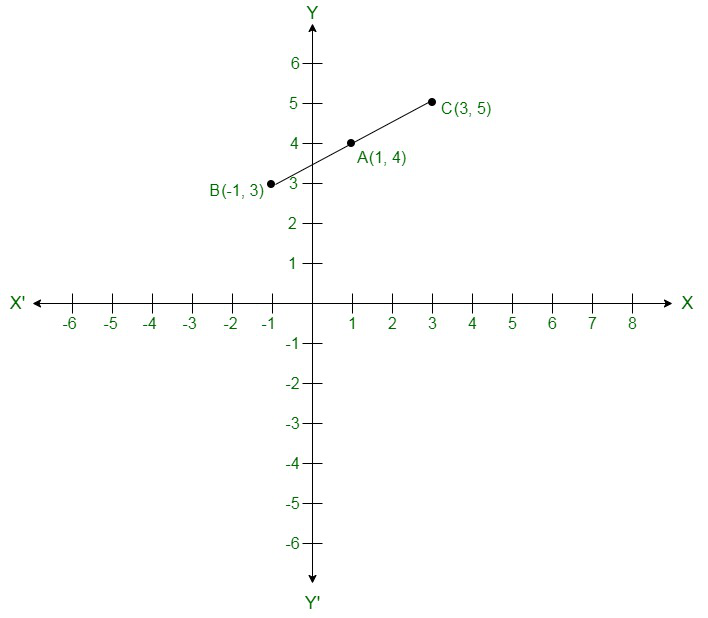As per the above graph: We can see that, Point A (1, 4) is already plotted on the graph, and a point of intersection of two intersecting lines.

Therefore, it is proved that the straight line passing through (3, 5) and (-1, 3) and also passes through A (1, 4).

### Question 8: From the choices given below, choose the equations whose graph is given in figure.

(i) y = x

(ii) x + y = 0

(iii) y = 2x

(iv) 2 + 3y = 7x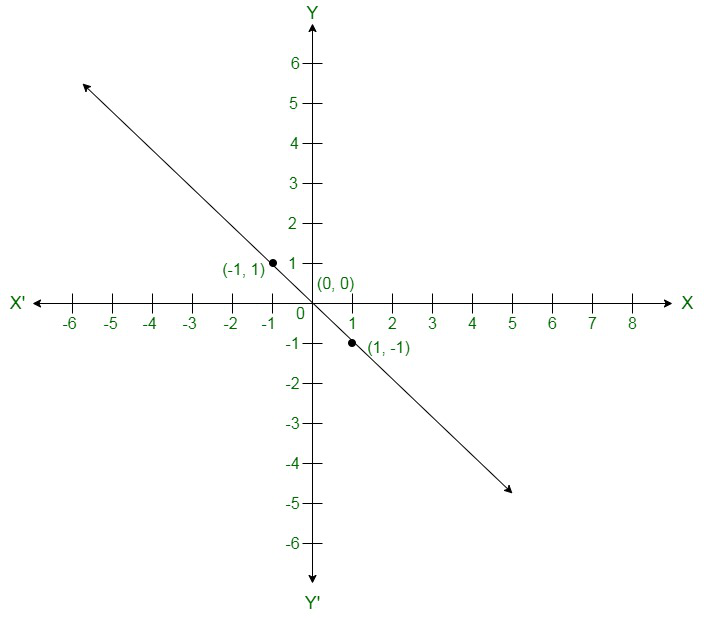Solution:

As per the given graph, co-ordinates (1, -1) and (-1, 1) are solutions of one of the equations.

Now, put the value of all the co-ordinates in each of the equation and verify which equation satisfy them.

(i) y = x

Put x = 1 and y = -1,

Thus, 1 ≠ -1

L.H.S ≠ R.H.S

By putting x = -1 and y = 1,

-1 ≠ 1

L.H.S ≠ R.H.S

Therefore,

y = x does not represent the graph in the given figure.

(ii) x + y = 0

Put x = 1 and y = -1,

⇒ 1 + (-1) = 0

⇒ 0 = 0

L.H.S = R.H.S

After putting x = -1 and y = 1,

(-1) + 1 = 0

0 = 0

L.H.S = R.H.S

Hence, the given solutions satisfy this equation.

(iii) y = 2x

Put x = 1 and y = -1

-1 = 2 (Can not be True)

Putting x = -1 and y = 1

1 = -2 (Can not be True)

Therefore, the given solutions does not satisfy this equation.

(iv) 2 + 3y = 7x

Put x = 1 and y = -1

2 – 3 = 7

-1 = 7 (Can not be true)

After putting x = -1 and y = 1

2 + 3 = -7

5 = -7 (Can not be True)

Therefore, the given solutions does not satisfy this equation.

### Question 9: From the choices given below, choose the equation whose graph is given fig:

(i) y = x + 2

(ii) y = x – 2

(iii) y = – x + 2

(iv) x + 2y = 6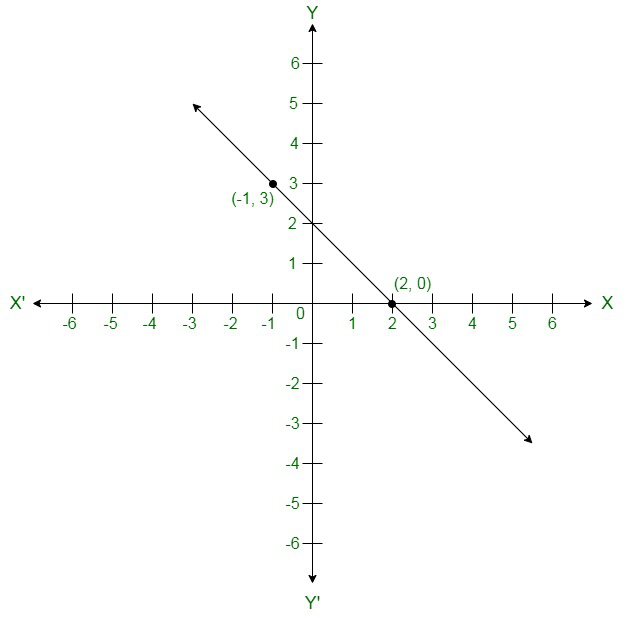Solution:

Given:

Here (-1, 3) and (2, 0) are the solution of one of the following given equations.

Verify that which of the equation satisfy both the points.

(i) y = x + 2

Put, x = -1 and y = 3

3 ≠ – 1 + 2

L.H.S ≠ R.H.S

By putting, x = 2 and y = 0

0 ≠ 4

L.H.S ≠ R.H.S

Hence, this solution does not satisfy the given equation.

(ii) y = x – 2

Put x = -1 and y = 3

3 ≠ – 1 – 2

L.H.S ≠ R.H.S

By putting, x = 2 and y = 0

0 = 0

L.H.S = R.H.S

Hence, the given solutions does not satisfy this equation completely.

(iii) y = – x + 2

Put x = – 1 and y = 3

3 = – (– 1) + 2

L.H.S = R.H.S

By putting x = 2 and y = 0

0 = -2 + 2

0 = 0

L.H.S = R.H.S

Thus, (0, 2) and (-1,3) satisfy this equation.

Therefore, this is the graph for equation y = -x + 2 .

(iv) x + 2y = 6

Put, x = – 1 and y = 3

-1 + 2(3) = 6

-1 + 6 = 6

5 = 6

L.H.S ≠ R.H.S

By putting x = 2 and y = 0

2 + 2(0) = 6

2 = 6

L.H.S ≠ R.H.S

Hence, this solution does not satisfy the given equation.

### Question 10: If the point (2, -2) lies on the graph of linear equation, 5x + ky = 4, find the value of k.

Solution:

Here point (2,-2) lies on the given linear equation, which implies (2, -2) satisfy this equation 5x + ky = 4.

So by putting x = 2 and y = -2 in 5x + ky = 4

5 × 2 + (-2) k = 4

10 – 2k = 4

2k = 10 – 4

2k = 6

k == 3

The value of k is 3.

My Personal Notes arrow_drop_up